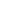User Rating: 5 / 5It seems that the FILTER() clause was introduced in SQL 2003 revision. The FILTER clause allows you to have a better control when doing statistics functions in SLQL such as COUNT(), MAX(), MIN(), SUM() and others. Sadly, so far only Postgresql has implemented it. According to my readings on the net, other big players such as MySQL, MariaDB, Oracle, Microsoft SQL, SQLite (and maybe others) do not support it. Lucky us, there is a way to translate those queries.

## Translating SUM(field) FILTER(WHERE) to SUM(CASE WHEN)

Please note this approach works with any other SQL function that returns a statistic calculation (MAX, MIN, AVERAGE). There is an alternative for COUNT(), I will write about it bellow.

Let's have this query as an example:

SELECT SUM(balance) FILTER (WHERE expired = 0) AS total FROM mytable

This could be translated as

SELECT SUM(CASE WHEN expired = 0 THEN balance ELSE 0 END) AS total FROM mytable

It is very important to put the ELSE 0, otherwise, the result could it be null (which it is different than zero). I know this example could be written in a very simple way. But trust me, there are many other SQL sentences that can hardly be translated into JOINS.

## Translating COUNT(*) FILTER(WHERE) to SUM(CASE WHEN)

There is no a direct way to support COUNT(CASE WHEN). At least, I tried on MariaDB 10.1 and I got a syntax error. There is another approach. Let us say we have this SQL sentence:

SELECT COUNT(*) FILTER (WHERE expired = 0) AS count FROM mytable

This could be translated as

SELECT SUM(CASE WHEN expired = 0 THEN 1 ELSE 0 END) AS count FROM mytable

We are changing the COUNT() into a SUM(), but instead of adding the value in the variable, we are fixing it with a 1.

Good luck!